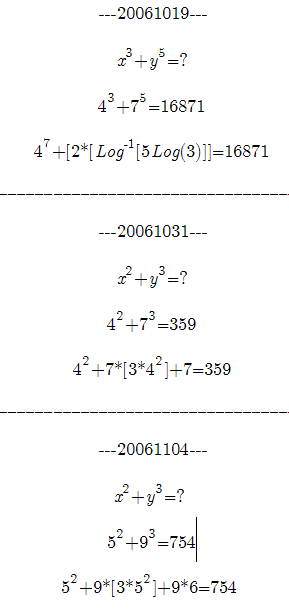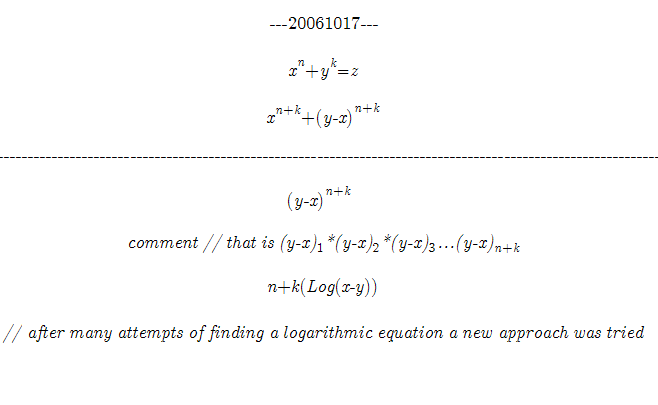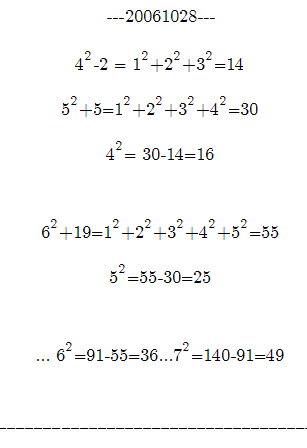Temporary Work _______ 20061103 Reading about logarithms makes one wonder if logarithms can perform multiplication and division and even simplify exponents. Why does the book say there is no rules for adding? We know this from basic algebra when studying exponents. In other words, x^2 + y^3 = ? . Now we realize this is a challenge to solve, but maybe solving the problem completely isn’t the goal. Maybe one of the variables x^2 or y^2 can be put into a simpler for of x or y. The question is, “is it possible to add two different variables that are exponential?” The hunch is yes. Yes that is at least to simplify them by removing one of the variables exponents. In fact we know it is possible. After all, if we know all the variables and exponents we would have no trouble finding the answer.This example is more than using factors. This is using the variables in a different way to calculate the answer. (There is both addition and multiplication here.) So it leads the mathematician to believe there is a series or pattern. The question is what is the pattern with different exponents and variables. (Don’t be confused. This example isn’t stating this is the correct pattern it just shows a pattern is possible.) The logarithmic work will be shown first. It is important to note that it is not correct. It is only to present the concept of adding variables and exponents. (20061029) This is no easy problem. It is said to be impossible by many math textbooks. It is “possible” it could be “impossible.” However some interesting things can be learned just by following a hunch. Here is a series of scratch work that will explain the attempts at finding a series among the exponents._______ 20061027 A parabola explains exponents. The key is finding the proportion between 2 numbers on there corresponding parabolas. It is much like adding or subtracting 2 parabolas to form a new one. It would be interesting to see how a quadratic equation is formed from a parabola. That is, the equation of the parabola. Hunch: Find the values of an exponent by the summation of the values of an involute. (See Chord vs. Circular Function) _______ 20061028 It would be interesting to see just what happens with the summation of the values under the graph of the parabola of the exponential function. If the exponent is know than so is the value of the parabola. For example the graphs of y = x^2 or y = x^3. Maybe there is a pattern.So far solution attempts look amateurish...like the steps are not following the fundamental rules. (This is true of the logarithmic equations which are not completely shown.) However on further inspection nothing could be further from the truth. Finding an quadratic equation from a parabola or using 2 parabolas (of certain exponential functions) to add x^2+y^3 is possible. Looking “amateurish” is ok at first. These are new ideas. It is meant to be a learning process. The problem has to start somewhere._______ 20061030 The next logical step after trying the integral is to use the rate of change or as it is known in calculus, the derivative. (20061104)— The derivative or rate of change is the key to putting x (in x^2+y^3) in terms of y so that they can be added or subtracted. _______ 20061104 The goal of adding x^2+y^3 is eliminating at least one of the variables exponent. We can do this by finding out how to add the smaller number to the larger with the smaller number value’s size described the same way as the larger value. In other words, the goal is to find how much x^2 is so much of y^3. Admittedly it is confusing, but hopefully the examples will show what is being attempted. And it will be shown how the derivative (rate of change) helps accomplish this. Show equation attempts — Note these attempts are not ready to share at this time. Note: At this time this is where the work stops. It is usually taught in class and textbooks that there is no rule for adding exponents. And it is considered an unsolved problem that may have no result that would be useful when solving equations. This is just an attempt to show how simple and fun it can be to work on an idea or concept. Approaching an unsolved problem is a challenging task, understated, but the things learned lead to other ideas. And it is a good warmup for other problems. It just might lead to great brainstorming sessions. _______ 20061105 It is important to note these examples and equations do not work for numbers greater than 10. (That is the first examples that were presented.) However, this should not discourage work! That is because this hunch is based on a parabola. The graphic representation of this problem is clear. It is summing the places of the exponential graph (a parabola) to form a new graph representing x^n+y^k. This is much like adding or manipulating the graph of a sine curve. Also note previously unsolved math has been posted on Constructor’s Corner. Sometimes it is unsolved. But some problems have been solved because the problem and data were presented in a way that helped organize problem solving efforts. So this information is placed here not as an answer but as a way to organize problem solving efforts. This is where the work stops on this document for now. I will continue to tinker with ideas. But this impossible problem is just one of many that can be worked on together on Constructor’s Corner. 8 people voted on a poll on Constructor’s Corner and expressed interest on working cooperatively on a math project. This is just a few to get started. 8 people are more than enough to solve problems and find some great answers. A Wiki where people can edit web pages and add content is on the way... But until then, May the Creative Force be with You